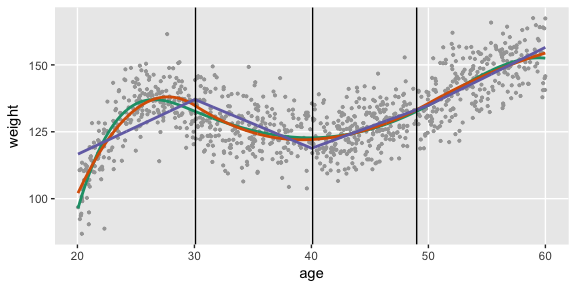# Simulating study data

#### 2018-05-11

Simulation using simstudy has two primary steps. First, the user defines the data elements of a data set. Second, the user generates the data, using the definitions in the first step. Additional functionality exists to simulate observed or randomized treatment assignment/exposures, to generate survival data, to create longitudinal/panel data, to create multi-level/hierarchical data, to create datasets with correlated variables based on a specified covariance structure, to merge datasets, to create data sets with missing data, and to create non-linear relationships with underlying spline curves.

## Defining the data

The key to simulating data in simstudy is the creation of series of data definition tables that look like this:

nr 7 0e+00 nonrandom identity
x1 10;20 0e+00 uniform identity
y1 nr + x1 * 2 8e+00 normal identity
y2 nr - 0.2 * x1 0e+00 poisson log
xnb nr - 0.2 * x1 5e-02 negBinomial log
xCat 0.3;0.2;0.5 0e+00 categorical identity
g1 5+xCat 1e+00 gamma log
a1 -3 + xCat 0e+00 binary logit
a2 -3 + xCat 1e+02 binomial logit

These definition tables can be generated two ways. One option is to to use any external editor that allows the creation of csv files, which can be read in with a call to defRead. An alternative is to make repeated calls to the function defData. Here, we illustrate the R code that builds this definition table internally:

def <- defData(varname = "nr", dist = "nonrandom", formula = 7, id = "idnum")
def <- defData(def, varname = "x1", dist = "uniform", formula = "10;20")
def <- defData(def, varname = "y1", formula = "nr + x1 * 2", variance = 8)
def <- defData(def, varname = "y2", dist = "poisson", formula = "nr - 0.2 * x1",
def <- defData(def, varname = "xnb", dist = "negBinomial", formula = "nr - 0.2 * x1",
variance = 0.05, link = "log")
def <- defData(def, varname = "xCat", formula = "0.3;0.2;0.5", dist = "categorical")
def <- defData(def, varname = "g1", dist = "gamma", formula = "5+xCat", variance = 1,
def <- defData(def, varname = "a1", dist = "binary", formula = "-3 + xCat",
def <- defData(def, varname = "a2", dist = "binomial", formula = "-3 + xCat",
variance = 100, link = "logit")

The first call to defData without specifying a definition name (in this example the definition name is def) creates a new data.table with a single row. An additional row is added to the table def each time the function defData is called. Each of these calls is the definition of a new field in the data set that will be generated. In this example, the first data field is named ‘nr’, defined as a constant with a value to be 7. In each call to defData the user defines a variable name, a distribution (the default is ‘normal’), a mean formula (if applicable), a variance parameter (if applicable), and a link function for the mean (defaults to ‘identity’).

The possible distributions include normal, gamma, poisson, zero-truncated poisson, negative binomial, binary, binomial, uniform, uniform integer, categorical, and deterministic/non-random. For all of these distributions, key parameters defining the distribution are entered in the formula, variance, and link fields.

In the case of the normal, gamma, and negative binomial distributions, the formula specifies the mean. The formula can be a scalar value (number) or a string that represents a function of previously defined variables in the data set definition (or, as we will see later, in a previously generated data set). In the example, the mean of y1, a normally distributed value, is declared as a linear function of nr and x1, and the mean of g1 is a function of the category defined by xCat. The variance field is defined only for normal and gamma random variables, and can only be defined as a scalar value. In the case of gamma random and negative binomial variables, the value entered in variance field is really a dispersion value $$d$$. The variance of a gamma distributed variable will be $$d \times mean^2$$, and for a negative binomial distributed variable, the variance will be $$mean + d*mean^2$$.

In the case of the poisson, zero-truncated poisson, and binary distributions, the formula also specifies the mean. The variance is not a valid parameter in these cases, but the link field is. The default link is ‘identity’ but a ‘log’ link is available for the Poisson distributions and a “logit” link is available for the binary outcomes. In this example, y2 is defined as Poisson random variable with a mean that is function of nr and x1 on the log scale. For binary variables, which take a value of 0 or 1, the formula represents probability (with the ‘identity’ link) or log odds (with the ‘logit’ link) of the variable having a value of 1. In the example, a1 has been defined as a binary random variable with a log odds that is a function of xCat.

In the case of the binomial distribution, the formula specifies the probability of success $$p$$, and the variance field is used to specify the number of trials $$n$$. The mean of this distribution is $$n*p$$, and the variance is $$n*p*(1-p)$$.

Variables defined with a uniform, uniform integer, categorical, or deterministic/non-random distribution are specified using the formula only. The variance and link fields are not used in these cases.

For a uniformly distributed variable, The formula is a string with the format “a;b”, where a and b are scalars or functions of previously defined variables. The uniform distribution has two parameters - the minimum and the maximum. In this case, a represents the minimum and b represents the maximum.

For a categorical variable with $$k$$ categories, the formula is a string of probabilities that sum to 1: “$$p_1 ; p_2 ; ... ; p_k$$”. $$p_1$$ is the probability of the random variable falling category 1, $$p_2$$ is the probability of category 2, etc. The probabilities can be specified as functions of other variables previously defined. In the example, xCat has three possibilities with probabilities 0.3, 0.2, and 0.5, respectively.

Non-random variables are defined by the formula. Since these variables are deterministic, variance is not relevant. They can be functions of previously defined variables or a scalar, as we see in the sample for variable defined as nr.

## Generating the data

After the data set definitions have been created, a new data set with $$n$$ observations can be created with a call to function genData. In this example, 1,000 observations are generated using the data set definitions in def, and then stored in the object dt:

dt <- genData(1000, def)
dt
##       idnum nr       x1       y1  y2 xnb xCat        g1 a1 a2
##    1:     1  7 18.71470 48.13110  25  36    3  882.3611  0 56
##    2:     2  7 12.63977 34.82680  87  97    3 1986.9499  1 51
##    3:     3  7 13.21247 34.96022  80  71    2 1460.2205  0 24
##    4:     4  7 19.21613 38.93975  17  16    1   77.6381  0 11
##    5:     5  7 10.70988 24.16021 148 110    2  696.4741  0 34
##   ---
##  996:   996  7 12.69114 34.43474  88 117    1  480.9245  1 13
##  997:   997  7 11.48129 31.34903 108 125    1  235.4808  0 11
##  998:   998  7 16.88184 41.60436  45  50    3 2425.0456  0 52
##  999:   999  7 10.24263 25.36589 151 107    3 2537.5048  0 46
## 1000:  1000  7 12.72076 33.53079  78  70    1  605.9685  0  8

New data can be added to an existing data set with a call to function addColumns. The new data definitions are created with a call to defData and then included as an argument in the call to addColumns:

addef <- defDataAdd(varname = "zExtra", dist = "normal", formula = "3 + y1",
variance = 2)

dt
##       idnum nr       x1       y1  y2 xnb xCat        g1 a1 a2   zExtra
##    1:     1  7 18.71470 48.13110  25  36    3  882.3611  0 56 52.13233
##    2:     2  7 12.63977 34.82680  87  97    3 1986.9499  1 51 37.48574
##    3:     3  7 13.21247 34.96022  80  71    2 1460.2205  0 24 34.65496
##    4:     4  7 19.21613 38.93975  17  16    1   77.6381  0 11 43.38695
##    5:     5  7 10.70988 24.16021 148 110    2  696.4741  0 34 26.82496
##   ---
##  996:   996  7 12.69114 34.43474  88 117    1  480.9245  1 13 36.27498
##  997:   997  7 11.48129 31.34903 108 125    1  235.4808  0 11 34.06526
##  998:   998  7 16.88184 41.60436  45  50    3 2425.0456  0 52 43.89020
##  999:   999  7 10.24263 25.36589 151 107    3 2537.5048  0 46 28.91570
## 1000:  1000  7 12.72076 33.53079  78  70    1  605.9685  0  8 35.01784

## Generating the treatment/exposure

Treatment assignment can be accomplished through the original data generation process, using defData and genData. However, the functions trtAssign and trtObserve provide more options to generate treatment assignment.

### Assigned treatment

Treatment assignment can simulate how treatment is made in a randomized study. Assignment to treatment groups can be (close to) balanced (as would occur in a block randomized trial); this balancing can be done without or without strata. Alternatively, the assignment can be left to chance without blocking; in this case, balance across treatment groups is not guaranteed, particularly with small sample sizes.

First, create the data definition:

def <- defData(varname = "male", dist = "binary", formula = 0.5, id = "cid")
def <- defData(def, varname = "over65", dist = "binary", formula = "-1.7 + .8*male",
def <- defData(def, varname = "baseDBP", dist = "normal", formula = 70, variance = 40)

dtstudy <- genData(330, def)

Balanced treatment assignment, stratified by gender and age category (not blood pressure)

study1 <- trtAssign(dtstudy, n = 3, balanced = TRUE, strata = c("male", "over65"),
grpName = "rxGrp")

study1
##      cid rxGrp male over65  baseDBP
##   1:   1     1    1      0 64.87188
##   2:   2     1    1      1 69.84233
##   3:   3     3    1      1 63.44874
##   4:   4     3    0      0 51.17674
##   5:   5     1    1      0 61.79753
##  ---
## 326: 326     2    1      1 65.16297
## 327: 327     1    1      1 80.57426
## 328: 328     1    0      0 61.29761
## 329: 329     3    0      0 65.35214
## 330: 330     1    0      0 69.88033

Balanced treatment assignment (without stratification)

study2 <- trtAssign(dtstudy, n = 3, balanced = TRUE, grpName = "rxGrp")

Random (unbalanced) treatment assignment

study3 <- trtAssign(dtstudy, n = 3, balanced = FALSE, grpName = "rxGrp")

Comparison of three treatment assignment mechanisms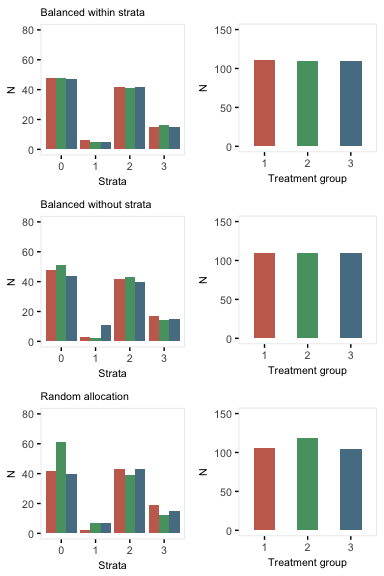### Observed treatment

If exposure or treatment is observed (rather than randomly assigned), use trtObserve to generate groups. There may be any number of possible exposure or treatment groups, and the probability of exposure to a specific level can depend on covariates already in the data set. In this case, there are three exposure groups that vary by gender and age:

formula1 <- c("-2 + 2*male - .5*over65", "-1 + 2*male + .5*over65")
dtExp <- trtObserve(dtstudy, formulas = formula1, logit.link = TRUE, grpName = "exposure")

Here are the exposure distributions by gender and age: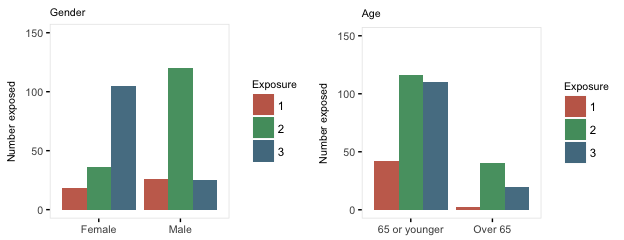Here is a second case of three exposures where the exposure is independent of any covariates. Note that specifying the formula as c(.35, .45) is the same as specifying it is c(.35, .45, .20). Also, when referring to probabilities, the identity link is used:

formula2 <- c(0.35, 0.45)

dtExp2 <- trtObserve(dtstudy, formulas = formula2, logit.link = FALSE, grpName = "exposure")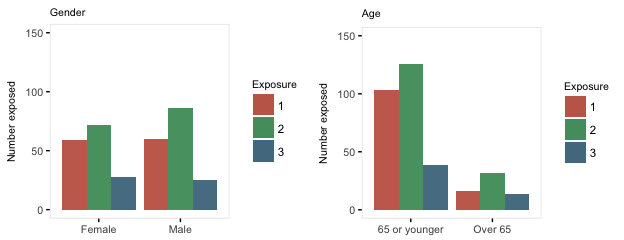## Survival data (updated 10-11-2017)

Time-to-event data, including both survival and censoring times, are created using functions defSurv and genSurv. The survival data definitions require a variable name as well as a specification of a scale value, which determines the mean survival time at a baseline level of covariates (i.e. all covariates set to 0). The Weibull distribution is used to generate these survival times. In addition, covariates (which have been defined previously) that influence survival time can be included in the formula field. Positive coefficients are associated with longer survival times (and lower hazard rates). Finally, the shape of the distribution can be specified. A shape value of 1 reflects the exponential distribution.

# Baseline data definitions

def <- defData(varname = "x1", formula = 0.5, dist = "binary")
def <- defData(def, varname = "x2", formula = 0.5, dist = "binary")
def <- defData(def, varname = "grp", formula = 0.5, dist = "binary")

# Survival data definitions

sdef <- defSurv(varname = "survTime", formula = "1.5*x1", scale = "grp*50 + (1-grp)*25",
shape = "grp*1 + (1-grp)*1.5")
sdef <- defSurv(sdef, varname = "censorTime", scale = 80, shape = 1)

sdef
##       varname formula               scale               shape
## 1:   survTime  1.5*x1 grp*50 + (1-grp)*25 grp*1 + (1-grp)*1.5
## 2: censorTime       0                  80                   1

The data are generated with calls to genData and genSurv:

# Baseline data definitions

dtSurv <- genData(300, def)
dtSurv <- genSurv(dtSurv, sdef)

head(dtSurv)
##    id x1 x2 grp survTime censorTime
## 1:  1  0  0   1   62.708     50.064
## 2:  2  1  0   0   22.040     93.278
## 3:  3  1  0   1    0.730     84.526
## 4:  4  1  0   1    1.523     39.144
## 5:  5  1  1   1    7.909     10.069
## 6:  6  0  0   1   10.652     16.670
# A comparison of survival by group and x1

dtSurv[, round(mean(survTime), 1), keyby = .(grp, x1)]
##    grp x1    V1
## 1:   0  0 202.3
## 2:   0  1  19.3
## 3:   1  0  46.9
## 4:   1  1   9.0

Observed survival times and censoring indicators can be generated by defining new fields:

cdef <- defDataAdd(varname = "obsTime", formula = "pmin(survTime, censorTime)",
dist = "nonrandom")
cdef <- defDataAdd(cdef, varname = "status", formula = "I(survTime <= censorTime)",
dist = "nonrandom")

head(dtSurv)
##    id x1 x2 grp survTime censorTime obsTime status
## 1:  1  0  0   1   62.708     50.064  50.064      0
## 2:  2  1  0   0   22.040     93.278  22.040      1
## 3:  3  1  0   1    0.730     84.526   0.730      1
## 4:  4  1  0   1    1.523     39.144   1.523      1
## 5:  5  1  1   1    7.909     10.069   7.909      1
## 6:  6  0  0   1   10.652     16.670  10.652      1
# estimate proportion of censoring by x1 and group

dtSurv[, round(1 - mean(status), 2), keyby = .(grp, x1)]
##    grp x1   V1
## 1:   0  0 0.55
## 2:   0  1 0.26
## 3:   1  0 0.38
## 4:   1  1 0.11

Here is a Kaplan-Meier plot of the data by the four groups: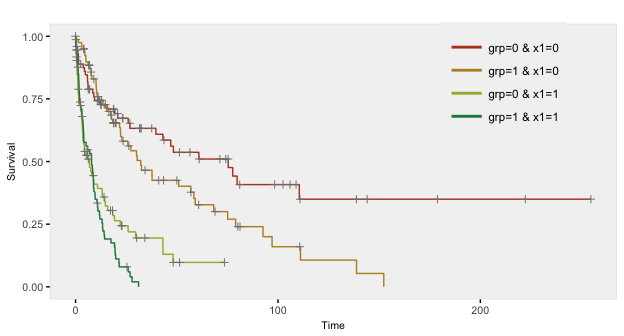## Longitudinal data

To simulate longitudinal data, we start with a ‘cross-sectional’ data set and convert it to a time-dependent data set. The original cross-sectional data set may or may not include time-dependent data in the columns. In the next example, we measure outcome Y once before and twice after intervention T in a randomized trial:

tdef <- defData(varname = "T", dist = "binary", formula = 0.5)
tdef <- defData(tdef, varname = "Y0", dist = "normal", formula = 10, variance = 1)
tdef <- defData(tdef, varname = "Y1", dist = "normal", formula = "Y0 + 5 + 5 * T",
variance = 1)
tdef <- defData(tdef, varname = "Y2", dist = "normal", formula = "Y0 + 10 + 5 * T",
variance = 1)

dtTrial <- genData(500, tdef)
dtTrial
##       id T        Y0       Y1       Y2
##   1:   1 1 10.302538 20.98756 25.15666
##   2:   2 1  9.342928 20.29140 24.80467
##   3:   3 0  9.141495 13.72892 18.20005
##   4:   4 1 10.529608 20.56103 26.01874
##   5:   5 1 11.219352 22.03580 24.45061
##  ---
## 496: 496 1  9.043124 16.90949 24.60409
## 497: 497 1 11.512700 20.86714 26.27011
## 498: 498 1  9.094696 18.33953 24.38047
## 499: 499 0 10.929032 16.15131 21.08117
## 500: 500 0 11.232353 16.49435 20.29298

The data in longitudinal form is created with a call to addPeriods. If the cross-sectional data includes time dependent data, then the number of periods nPeriods must be the same as the number of time dependent columns. If a variable is not declared as one of the timevars, it will be repeated each time period. In this example, the treatment indicator T is not specified as a time dependent variable. (Note: if there are two time-dependent variables, it is best to create two data sets and merge them. This will be shown later in the vignette).

dtTime <- addPeriods(dtTrial, nPeriods = 3, idvars = "id", timevars = c("Y0",
"Y1", "Y2"), timevarName = "Y")
dtTime
##        id period T         Y timeID
##    1:   1      0 1 10.302538      1
##    2:   1      1 1 20.987560      2
##    3:   1      2 1 25.156655      3
##    4:   2      0 1  9.342928      4
##    5:   2      1 1 20.291398      5
##   ---
## 1496: 499      1 0 16.151314   1496
## 1497: 499      2 0 21.081167   1497
## 1498: 500      0 0 11.232353   1498
## 1499: 500      1 0 16.494354   1499
## 1500: 500      2 0 20.292984   1500

This is what the longitudinal data look like: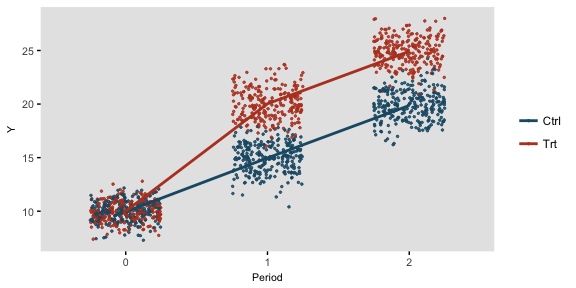### Longitudinal data with varying observation and interval times

It is also possible to generate longitudinal data with varying numbers of measurement periods as well as varying time intervals between each measurement period. This is done by defining specific variables in the data set that define the number of observations per subject and the average interval time between each observation. nCount defines the number of measurements for an individual; mInterval specifies the average time between intervals for an subject; and vInterval specifies the variance of those interval times. If vInterval is set to 0 or is not defined, the interval for a subject is determined entirely by the mean interval. If vInterval is greater than 0, time intervals are generated using a gamma distribution with mean and dispersion specified.

In this simple example, the cross-sectional data generates individuals with a different number of measurement observations and different times between each observation. Data for two of these individuals is printed:

def <- defData(varname = "xbase", dist = "normal", formula = 20, variance = 3)
def <- defData(def, varname = "nCount", dist = "noZeroPoisson", formula = 6)
def <- defData(def, varname = "mInterval", dist = "gamma", formula = 30, variance = 0.01)
def <- defData(def, varname = "vInterval", dist = "nonrandom", formula = 0.07)

dt <- genData(200, def)
dt[id %in% c(8, 121)]  # View individuals 8 and 121
##     id    xbase nCount mInterval vInterval
## 1:   8 20.56345      4  27.26486      0.07
## 2: 121 18.21229      4  30.69029      0.07

The resulting longitudinal data for these two subjects can be inspected after a call to addPeriods. Notice that no parameters need to be set since all information resides in the data set itself:

dtPeriod <- addPeriods(dt)
dtPeriod[id %in% c(8, 121)]  # View individuals 8 and 121 only
##     id period    xbase time timeID
## 1:   8      0 20.56345    0     37
## 2:   8      1 20.56345   33     38
## 3:   8      2 20.56345   64     39
## 4:   8      3 20.56345   87     40
## 5: 121      0 18.21229    0    750
## 6: 121      1 18.21229   37    751
## 7: 121      2 18.21229   62    752
## 8: 121      3 18.21229   93    753

If a time sensitive measurement is added to the data set …

def2 <- defDataAdd(varname = "Y", dist = "normal", formula = "15 + .1 * time",
variance = 5)
dtPeriod <- addColumns(def2, dtPeriod)

… a plot of a five randomly selected individuals looks like this: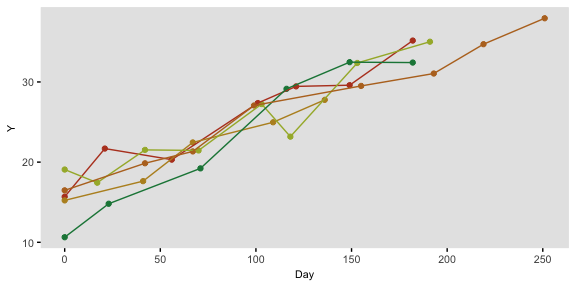## Clustered data

The function genCluster generates multilevel or clustered data based on a previously generated data set that is one “level” up from the clustered data. For example, if there is a data set that contains school level (considered here to be level 2), classrooms (level 1) can be generated. And then, students (now level 1) can be generated within classrooms (now level 2)

In the example here, we do in fact generate school, class, and student level data. There are eight schools, four of which are randomized to receive an intervention. The number of classes per school varies, as does the number of students per class. (It is straightforward to generate fully balanced data by using constant values.) The outcome of interest is a test score, which is influenced by gender and the intervention. In addition, test scores vary by schools, and by classrooms, so the simulation provides random effects at each of these levels.

We start by defining the school level data:

gen.school <- defData(varname = "s0", dist = "normal", formula = 0, variance = 3,
id = "idSchool")
gen.school <- defData(gen.school, varname = "nClasses", dist = "noZeroPoisson",
formula = 3)

dtSchool <- genData(8, gen.school)
dtSchool <- trtAssign(dtSchool, n = 2)

dtSchool
##    idSchool trtGrp         s0 nClasses
## 1:        1      1  0.9952972        1
## 2:        2      0  1.1190035        2
## 3:        3      0 -2.9065851        1
## 4:        4      1  0.3288244        3
## 5:        5      1  2.6920645        2
## 6:        6      0  2.2788338        5
## 7:        7      1 -1.7853013        1
## 8:        8      0 -1.0547836        2

The classroom level data are generated with a call to genCluster, and then school level data is added by a call to addColumns:

gen.class <- defDataAdd(varname = "c0", dist = "normal", formula = 0, variance = 2)
gen.class <- defDataAdd(gen.class, varname = "nStudents", dist = "noZeroPoisson",
formula = 20)

dtClass <- genCluster(dtSchool, "idSchool", numIndsVar = "nClasses", level1ID = "idClass")

head(dtClass, 10)
##     idSchool trtGrp         s0 nClasses idClass          c0 nStudents
##  1:        1      1  0.9952972        1       1 -0.45176838        20
##  2:        2      0  1.1190035        2       2 -0.13200400        21
##  3:        2      0  1.1190035        2       3 -0.07759884        24
##  4:        3      0 -2.9065851        1       4 -1.49094361        16
##  5:        4      1  0.3288244        3       5 -0.51324530        13
##  6:        4      1  0.3288244        3       6  0.37462222        20
##  7:        4      1  0.3288244        3       7  0.28395738        22
##  8:        5      1  2.6920645        2       8 -0.65913372        19
##  9:        5      1  2.6920645        2       9 -2.47419748        10
## 10:        6      0  2.2788338        5      10  0.92076842        25

Finally, the student level data are added using the same process:

gen.student <- defDataAdd(varname = "Male", dist = "binary",
formula = 0.5)
gen.student <- defDataAdd(gen.student, varname = "age", dist = "uniform",
formula = "9.5; 10.5")
gen.student <- defDataAdd(gen.student, varname = "test", dist = "normal",
formula = "50 - 5*Male + s0 + c0 + 8 * trtGrp", variance = 2)
dtStudent <- genCluster(dtClass, cLevelVar = "idClass", numIndsVar = "nStudents",
level1ID = "idChild")

dtStudent <- addColumns(gen.student, dtStudent)

This is what the clustered data look like. Each classroom is represented by a box, and each school is represented by a color. The intervention group is highlighted by dark outlines: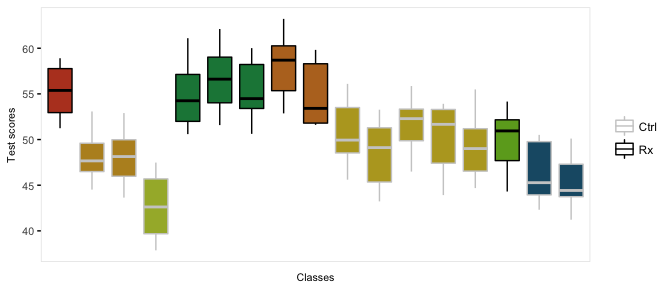## Correlated data

Sometimes it is desirable to simulate correlated data from a correlation matrix directly. For example, a simulation might require two random effects (e.g. a random intercept and a random slope). Correlated data like this could be generated using the defData functionality, but it may be more natural to do this with genCorData or addCorData. Currently, simstudy can only generate multivariate normal using these functions. (In the future, additional distributions will be available.)

genCorData requires the user to specify a mean vector mu, a single standard deviation or a vector of standard deviations sigma, and either a correlation matrix corMatrix or a correlation coefficient rho and a correlation structure corsrt. It is easy to see how this can be used from a few different examples.

# specifying a specific correlation matrix C
C <- matrix(c(1, 0.7, 0.2, 0.7, 1, 0.8, 0.2, 0.8, 1), nrow = 3)
C
##      [,1] [,2] [,3]
## [1,]  1.0  0.7  0.2
## [2,]  0.7  1.0  0.8
## [3,]  0.2  0.8  1.0
# generate 3 correlated variables with different location and scale for each
# field
dt <- genCorData(1000, mu = c(4, 12, 3), sigma = c(1, 2, 3), corMatrix = C)
dt
##         id       V1        V2        V3
##    1:    1 2.371760  7.267119 -3.353150
##    2:    2 5.338586 12.025703 -0.221679
##    3:    3 4.540329 11.572486  1.527022
##    4:    4 4.296518 12.982947  3.847468
##    5:    5 4.227715 14.176033  6.355379
##   ---
##  996:  996 5.413130 12.897643  2.053842
##  997:  997 3.747452 13.019754  6.195115
##  998:  998 4.713292 11.215560  1.966497
##  999:  999 4.289476 13.696805  6.402623
## 1000: 1000 5.307649 11.702413  1.066196
# estimate correlation matrix
dt[, round(cor(cbind(V1, V2, V3)), 1)]
##     V1  V2  V3
## V1 1.0 0.7 0.3
## V2 0.7 1.0 0.8
## V3 0.3 0.8 1.0
# estimate standard deviation
dt[, round(sqrt(diag(var(cbind(V1, V2, V3)))), 1)]
## V1 V2 V3
##  1  2  3
# generate 3 correlated variables with different location but same standard
# deviation and compound symmetry (cs) correlation matrix with correlation
# coefficient = 0.4.  Other correlation matrix structures are 'independent'
# ('ind') and 'auto-regressive' ('ar1').

dt <- genCorData(1000, mu = c(4, 12, 3), sigma = 3, rho = 0.4, corstr = "cs",
cnames = c("x0", "x1", "x2"))
dt
##         id        x0        x1         x2
##    1:    1 5.5796361 14.599671  1.9399777
##    2:    2 1.6786843 14.202972  2.9523835
##    3:    3 1.4593530  6.326193  1.5974447
##    4:    4 8.9378636 11.414865  2.5973158
##    5:    5 8.9159529 15.405254  4.8266588
##   ---
##  996:  996 4.5926670 12.705928 -0.2350507
##  997:  997 0.9501757 11.326906  2.1582591
##  998:  998 4.4282839 14.203071  1.4537402
##  999:  999 7.0764204 14.810984  5.0886760
## 1000: 1000 5.3806136  7.791936  4.4242018
# estimate correlation matrix
dt[, round(cor(cbind(x0, x1, x2)), 1)]
##     x0  x1  x2
## x0 1.0 0.4 0.4
## x1 0.4 1.0 0.4
## x2 0.4 0.4 1.0
# estimate standard deviation
dt[, round(sqrt(diag(var(cbind(x0, x1, x2)))), 1)]
## x0 x1 x2
##  3  3  3

The new data generated by genCorData can be merged with an existing data set. Alternatively, addCorData will do this directly:

# define and generate the original data set
def <- defData(varname = "x", dist = "normal", formula = 0, variance = 1, id = "cid")
dt <- genData(1000, def)

# add new correlate fields a0 and a1 to 'dt'
dt <- addCorData(dt, idname = "cid", mu = c(0, 0), sigma = c(2, 0.2), rho = -0.2,
corstr = "cs", cnames = c("a0", "a1"))

dt
##        cid          x         a0          a1
##    1:    1 -2.5176420 -1.6711502  0.18836912
##    2:    2 -1.1643167 -0.8355326  0.02117165
##    3:    3  0.2246621 -3.4330778  0.08707207
##    4:    4 -0.1207224  1.5254125 -0.14845140
##    5:    5 -0.4324263 -2.1750978 -0.14895895
##   ---
##  996:  996  0.0213324 -0.1119475 -0.14549240
##  997:  997 -0.7639465 -2.8598024 -0.34985504
##  998:  998  1.0565218 -0.1728586  0.05928381
##  999:  999 -1.9819216 -2.0273570 -0.14192394
## 1000: 1000  1.0946993  0.3730754  0.10098404
# estimate correlation matrix
dt[, round(cor(cbind(a0, a1)), 1)]
##      a0   a1
## a0  1.0 -0.1
## a1 -0.1  1.0
# estimate standard deviation
dt[, round(sqrt(diag(var(cbind(a0, a1)))), 1)]
##  a0  a1
## 2.1 0.2

Two additional functions facilitate the generation of correlated data from binomial, poisson, gamma, and uniform distributions: genCorGen and addCorGen.

genCorGen is an extension of genCorData. In the first example, we are generating data from a multivariate Poisson distribution. We start by specifying the mean of the Poisson distribution for each new variable, and then we specify the correlation structure, just as we did with the normal distribution.

l <- c(8, 10, 12)  # lambda for each new variable

dx <- genCorGen(1000, nvars = 3, params1 = l, dist = "poisson", rho = 0.3, corstr = "cs",
wide = TRUE)
dx
##         id V1 V2 V3
##    1:    1  8 13 19
##    2:    2  7  8 13
##    3:    3  5  9 10
##    4:    4 12 13 14
##    5:    5  8  7 13
##   ---
##  996:  996  9 10 10
##  997:  997  7 10 10
##  998:  998  7 11  7
##  999:  999  3  9 12
## 1000: 1000  6  8 10
round(cor(as.matrix(dx[, .(V1, V2, V3)])), 2)
##      V1   V2   V3
## V1 1.00 0.26 0.28
## V2 0.26 1.00 0.25
## V3 0.28 0.25 1.00

We can also generate correlated binary data by specifying the probabilities:

genCorGen(1000, nvars = 3, params1 = c(0.3, 0.5, 0.7), dist = "binary", rho = 0.8,
corstr = "cs", wide = TRUE)
##         id V1 V2 V3
##    1:    1  1  1  1
##    2:    2  0  0  1
##    3:    3  0  0  0
##    4:    4  0  1  0
##    5:    5  1  1  1
##   ---
##  996:  996  1  1  1
##  997:  997  0  0  0
##  998:  998  0  0  1
##  999:  999  0  1  1
## 1000: 1000  0  1  1

The gamma distribution requires two parameters - the mean and dispersion. (These are converted into shape and rate parameters more commonly used.)

dx <- genCorGen(1000, nvars = 3, params1 = l, params2 = c(1, 1, 1), dist = "gamma",
rho = 0.7, corstr = "cs", wide = TRUE, cnames = "a, b, c")
dx
##         id         a          b          c
##    1:    1  3.140608  4.3007520  2.7383612
##    2:    2 10.307179 20.0914900 40.3768023
##    3:    3  0.419335  2.5567801  8.4867149
##    4:    4  1.716652  0.7270978  0.3026686
##    5:    5  5.368087  5.1906768 11.9544632
##   ---
##  996:  996  8.891153 21.4953250  9.0533682
##  997:  997  6.062700 24.5878581 32.2937064
##  998:  998  1.116576  1.7668121  2.7228186
##  999:  999  7.378934 21.0885475 24.7596103
## 1000: 1000 38.303642 64.8877476 47.2871971
round(cor(as.matrix(dx[, .(a, b, c)])), 2)
##      a    b    c
## a 1.00 0.64 0.62
## b 0.64 1.00 0.62
## c 0.62 0.62 1.00

These data sets can be generated in either wide or long form. So far, we have generated wide form data, where there is one row per unique id. Now, we will generate data using the long form, where the correlated data are on different rows, so that there are repeated measurements for each id. An id will have multiple records (i.e. one id will appear on multiple rows):

dx <- genCorGen(1000, nvars = 3, params1 = l, params2 = c(1, 1, 1), dist = "gamma",
rho = 0.7, corstr = "cs", wide = FALSE, cnames = "NewCol")
dx
##         id period     NewCol
##    1:    1      0  0.7224226
##    2:    1      1  0.1139402
##    3:    1      2  0.4282428
##    4:    2      0  5.8594956
##    5:    2      1  3.4056995
##   ---
## 2996:  999      1  2.0735110
## 2997:  999      2  0.6108214
## 2998: 1000      0 12.6843514
## 2999: 1000      1 10.3532289
## 3000: 1000      2 19.6547040

addCorGen allows us to create correlated data from an existing data set, as one can already do using addCorData. In the case of addCorGen, the parameter(s) used to define the distribution are created as a field (or fields) in the dataset. The correlated data are added to the existing data set. In the example below, we are going to generate three sets (poisson, binary, and gamma) of correlated data with means that are a function of the variable xbase, which varies by id.

First we define the data and generate a data set:

def <- defData(varname = "xbase", formula = 5, variance = 0.2, dist = "gamma",
id = "cid")
def <- defData(def, varname = "lambda", formula = ".5 + .1*xbase", dist = "nonrandom",
def <- defData(def, varname = "p", formula = "-2 + .3*xbase", dist = "nonrandom",
def <- defData(def, varname = "gammaMu", formula = ".5 + .2*xbase", dist = "nonrandom",
def <- defData(def, varname = "gammaDis", formula = 1, dist = "nonrandom")

dt <- genData(10000, def)
dt
##          cid    xbase   lambda         p  gammaMu gammaDis
##     1:     1 4.778931 2.658848 0.3620862 4.287853        1
##     2:     2 5.808020 2.947042 0.4359553 5.267754        1
##     3:     3 7.691522 3.557835 0.5762643 7.677580        1
##     4:     4 4.172825 2.502481 0.3212240 3.798343        1
##     5:     5 2.805151 2.182596 0.2389482 2.889346        1
##    ---
##  9996:  9996 2.945426 2.213428 0.2466848 2.971555        1
##  9997:  9997 3.695096 2.385741 0.2908063 3.452226        1
##  9998:  9998 5.112397 2.749007 0.3854969 4.583576        1
##  9999:  9999 4.407292 2.561849 0.3367497 3.980703        1
## 10000: 10000 3.028564 2.231907 0.2513489 3.021377        1

The Poisson distribution has a single parameter, lambda:

dtX1 <- addCorGen(dtOld = dt, idvar = "cid", nvars = 3, rho = 0.1, corstr = "cs",
dist = "poisson", param1 = "lambda", cnames = "a, b, c")
dtX1
##          cid    xbase   lambda         p  gammaMu gammaDis a b c
##     1:     1 4.778931 2.658848 0.3620862 4.287853        1 1 0 3
##     2:     2 5.808020 2.947042 0.4359553 5.267754        1 2 1 2
##     3:     3 7.691522 3.557835 0.5762643 7.677580        1 5 1 3
##     4:     4 4.172825 2.502481 0.3212240 3.798343        1 2 3 3
##     5:     5 2.805151 2.182596 0.2389482 2.889346        1 1 2 2
##    ---
##  9996:  9996 2.945426 2.213428 0.2466848 2.971555        1 1 0 1
##  9997:  9997 3.695096 2.385741 0.2908063 3.452226        1 2 5 3
##  9998:  9998 5.112397 2.749007 0.3854969 4.583576        1 2 3 2
##  9999:  9999 4.407292 2.561849 0.3367497 3.980703        1 5 1 3
## 10000: 10000 3.028564 2.231907 0.2513489 3.021377        1 2 2 1

The Bernoulli (binary) distribution has a single parameter, p:

dtX2 <- addCorGen(dtOld = dt, idvar = "cid", nvars = 4, rho = 0.4, corstr = "ar1",
dist = "binary", param1 = "p")
dtX2
##          cid    xbase   lambda         p  gammaMu gammaDis V1 V2 V3 V4
##     1:     1 4.778931 2.658848 0.3620862 4.287853        1  1  1  0  0
##     2:     2 5.808020 2.947042 0.4359553 5.267754        1  1  1  1  1
##     3:     3 7.691522 3.557835 0.5762643 7.677580        1  1  0  0  0
##     4:     4 4.172825 2.502481 0.3212240 3.798343        1  0  0  0  1
##     5:     5 2.805151 2.182596 0.2389482 2.889346        1  0  1  0  0
##    ---
##  9996:  9996 2.945426 2.213428 0.2466848 2.971555        1  0  1  0  1
##  9997:  9997 3.695096 2.385741 0.2908063 3.452226        1  0  0  0  0
##  9998:  9998 5.112397 2.749007 0.3854969 4.583576        1  0  1  1  0
##  9999:  9999 4.407292 2.561849 0.3367497 3.980703        1  0  1  0  1
## 10000: 10000 3.028564 2.231907 0.2513489 3.021377        1  1  0  0  1

The Gamma distribution has two parameters - in simstudy the mean and dispersion are specified:

dtX3 <- addCorGen(dtOld = dt, idvar = "cid", nvars = 4, rho = 0.4, corstr = "cs",
dist = "gamma", param1 = "gammaMu", param2 = "gammaDis")
dtX3
##          cid    xbase   lambda         p  gammaMu gammaDis          V1
##     1:     1 4.778931 2.658848 0.3620862 4.287853        1  4.97177856
##     2:     2 5.808020 2.947042 0.4359553 5.267754        1  2.26479852
##     3:     3 7.691522 3.557835 0.5762643 7.677580        1  0.12458910
##     4:     4 4.172825 2.502481 0.3212240 3.798343        1  7.81251102
##     5:     5 2.805151 2.182596 0.2389482 2.889346        1  1.62216482
##    ---
##  9996:  9996 2.945426 2.213428 0.2466848 2.971555        1  4.24746781
##  9997:  9997 3.695096 2.385741 0.2908063 3.452226        1  5.70327045
##  9998:  9998 5.112397 2.749007 0.3854969 4.583576        1 35.38132755
##  9999:  9999 4.407292 2.561849 0.3367497 3.980703        1  2.74207523
## 10000: 10000 3.028564 2.231907 0.2513489 3.021377        1  0.06424847
##                 V2         V3         V4
##     1:  1.40906241 0.20945217  4.5779254
##     2:  2.71007560 5.79073106  0.9995026
##     3:  0.43022945 2.01899375  0.6515874
##     4:  3.04618317 3.10408320 10.5024364
##     5:  0.68578948 5.88514410  4.6718322
##    ---
##  9996:  8.59012825 3.04698214  4.4227683
##  9997:  3.80080948 3.01075330  0.1742112
##  9998: 11.06796032 9.11689081  7.0568069
##  9999:  0.06362115 1.17219267  4.9186347
## 10000:  0.41277138 0.01914079  0.7319734

If we have data in long form (e.g. longitudinal data), the function will recognize the structure:

def <- defData(varname = "xbase", formula = 5, variance = 0.4, dist = "gamma",
id = "cid")
def <- defData(def, "nperiods", formula = 3, dist = "noZeroPoisson")

def2 <- defDataAdd(varname = "lambda", formula = ".5+.5*period + .1*xbase",
dist = "nonrandom", link = "log")

dt <- genData(1000, def)

dtLong <- addPeriods(dt, idvars = "cid", nPeriods = 3)

dtLong
##        cid period     xbase nperiods timeID    lambda
##    1:    1      0 10.957824        2      1  4.932187
##    2:    1      1 10.957824        2      2  8.131801
##    3:    1      2 10.957824        2      3 13.407073
##    4:    2      0  1.322296        1      4  1.881802
##    5:    2      1  1.322296        1      5  3.102566
##   ---
## 2996:  999      1  5.089007        2   2996  4.521757
## 2997:  999      2  5.089007        2   2997  7.455118
## 2998: 1000      0  1.856730        1   2998  1.985107
## 2999: 1000      1  1.856730        1   2999  3.272889
## 3000: 1000      2  1.856730        1   3000  5.396081
### Generate the data

dtX3 <- addCorGen(dtOld = dtLong, idvar = "cid", nvars = 3, rho = 0.6, corstr = "cs",
dist = "poisson", param1 = "lambda", cnames = "NewPois")
dtX3
##        cid period     xbase nperiods timeID    lambda NewPois
##    1:    1      0 10.957824        2      1  4.932187       3
##    2:    1      1 10.957824        2      2  8.131801       9
##    3:    1      2 10.957824        2      3 13.407073      14
##    4:    2      0  1.322296        1      4  1.881802       2
##    5:    2      1  1.322296        1      5  3.102566       3
##   ---
## 2996:  999      1  5.089007        2   2996  4.521757       2
## 2997:  999      2  5.089007        2   2997  7.455118       5
## 2998: 1000      0  1.856730        1   2998  1.985107       3
## 2999: 1000      1  1.856730        1   2999  3.272889       5
## 3000: 1000      2  1.856730        1   3000  5.396081       8

We can fit a generalized estimating equation (GEE) model and examine the coefficients and the working correlation matrix. They match closely to the data generating parameters:

geefit <- gee(NewPois ~ period + xbase, data = dtX3, id = cid, family = poisson,
corstr = "exchangeable")
## Beginning Cgee S-function, @(#) geeformula.q 4.13 98/01/27
## running glm to get initial regression estimate
## (Intercept)      period       xbase
##   0.4901460   0.4980868   0.1007985
round(summary(geefit)$working.correlation, 2) ## [,1] [,2] [,3] ## [1,] 1.00 0.59 0.59 ## [2,] 0.59 1.00 0.59 ## [3,] 0.59 0.59 1.00 ## Missing data After generating a complete data set, it is possible to generate missing data. defMiss defines the parameters of missingness. genMiss generates a missing data matrix of indicators for each field. Indicators are set to 1 if the data are missing for a subject, 0 otherwise. genObs creates a data set that reflects what would have been observed had data been missing; this is a replicate of the original data set with “NAs” replacing values where missing data has been generated. By controlling the parameters of missingness, it is possible to represent different missing data mechanisms: (1) missing completely at random (MCAR), where the probability missing data is independent of any covariates, measured or unmeasured, that are associated with the measure, (2) missing at random (MAR), where the probability of subject missing data is a function only of observed covariates that are associated with the measure, and (3) not missing at random (NMAR), where the probability of missing data is related to unmeasured covariates that are associated with measure. These possibilities are illustrated with an example. A data set of 1000 observations with three “outcome” measures" x1, x2, and x3 is defined. This data set also includes two independent predictors, m and u that largely determine the value of each outcome (subject to random noise). def1 <- defData(varname = "m", dist = "binary", formula = 0.5) def1 <- defData(def1, "u", dist = "binary", formula = 0.5) def1 <- defData(def1, "x1", dist = "normal", formula = "20*m + 20*u", variance = 2) def1 <- defData(def1, "x2", dist = "normal", formula = "20*m + 20*u", variance = 2) def1 <- defData(def1, "x3", dist = "normal", formula = "20*m + 20*u", variance = 2) dtAct <- genData(1000, def1) In this example, the missing data mechanism is different for each outcome. As defined below, missingness for x1 is MCAR, since the probability of missing is fixed. Missingness for x2 is MAR, since missingness is a function of m, a measured predictor of x2. And missingness for x3 is NMAR, since the probability of missing is dependent on u, an unmeasured predictor of x3: defM <- defMiss(varname = "x1", formula = 0.15, logit.link = FALSE) defM <- defMiss(defM, varname = "x2", formula = ".05 + m * 0.25", logit.link = FALSE) defM <- defMiss(defM, varname = "x3", formula = ".05 + u * 0.25", logit.link = FALSE) defM <- defMiss(defM, varname = "u", formula = 1, logit.link = FALSE) # not observed missMat <- genMiss(dtName = dtAct, missDefs = defM, idvars = "id") dtObs <- genObs(dtAct, missMat, idvars = "id") missMat ## id x1 x2 x3 u m ## 1: 1 0 0 0 1 0 ## 2: 2 0 0 0 1 0 ## 3: 3 0 0 0 1 0 ## 4: 4 0 0 0 1 0 ## 5: 5 0 0 0 1 0 ## --- ## 996: 996 0 0 0 1 0 ## 997: 997 1 0 0 1 0 ## 998: 998 0 0 0 1 0 ## 999: 999 1 0 0 1 0 ## 1000: 1000 0 1 1 1 0 dtObs ## id m u x1 x2 x3 ## 1: 1 1 NA 21.2386093 20.3915020 21.320315 ## 2: 2 1 NA 20.3091784 19.5610545 22.097368 ## 3: 3 1 NA 21.3622296 15.5862973 20.275298 ## 4: 4 0 NA -0.0905736 1.3907574 -1.215204 ## 5: 5 0 NA -0.7610461 -0.2431899 1.850877 ## --- ## 996: 996 1 NA 42.4703773 40.6428296 39.991157 ## 997: 997 1 NA NA 39.7122805 41.226036 ## 998: 998 1 NA 19.9300903 19.8683944 20.618006 ## 999: 999 0 NA NA 17.5998314 21.615767 ## 1000: 1000 1 NA 40.6976095 NA NA The impacts of the various data mechanisms on estimation can be seen with a simple calculation of means using both the “true” data set without missing data as a comparison for the “observed” data set. Since x1 is MCAR, the averages for both data sets are roughly equivalent. However, we can see below that estimates for x2 and x3 are biased, as the difference between observed and actual is not close to 0: # Two functions to calculate means and compare them rmean <- function(var, digits = 1) { round(mean(var, na.rm = TRUE), digits) } showDif <- function(dt1, dt2, rowName = c("Actual", "Observed", "Difference")) { dt <- data.frame(rbind(dt1, dt2, dt1 - dt2)) rownames(dt) <- rowName return(dt) } # data.table functionality to estimate means for each data set meanAct <- dtAct[, .(x1 = rmean(x1), x2 = rmean(x2), x3 = rmean(x3))] meanObs <- dtObs[, .(x1 = rmean(x1), x2 = rmean(x2), x3 = rmean(x3))] showDif(meanAct, meanObs) ## x1 x2 x3 ## Actual 19.9 19.8 19.8 ## Observed 20.1 18.4 18.5 ## Difference -0.2 1.4 1.3 After adjusting for the measured covariate m, the bias for the estimate of the mean of x2 is mitigated, but not for x3, since u is not observed: meanActm <- dtAct[, .(x1 = rmean(x1), x2 = rmean(x2), x3 = rmean(x3)), keyby = m] meanObsm <- dtObs[, .(x1 = rmean(x1), x2 = rmean(x2), x3 = rmean(x3)), keyby = m] # compare observed and actual when m = 0 showDif(meanActm[m == 0, .(x1, x2, x3)], meanObsm[m == 0, .(x1, x2, x3)]) ## x1 x2 x3 ## Actual 9.5 9.4 9.3 ## Observed 9.5 9.3 8.1 ## Difference 0.0 0.1 1.2 # compare observed and actual when m = 1 showDif(meanActm[m == 1, .(x1, x2, x3)], meanObsm[m == 1, .(x1, x2, x3)]) ## x1 x2 x3 ## Actual 29.3 29.3 29.2 ## Observed 29.3 29.4 27.9 ## Difference 0.0 -0.1 1.3 ## Longitudinal data with missingness Missingness can occur, of course, in the context of longitudinal data. missDef provides two additional arguments that are relevant for these types of data: baseline and monotonic. In the case of variables that are measured at baseline only, a missing value would be reflected throughout the course of the study. In the case where a variable is time-dependent (i.e it is measured at each time point), it is possible to declare missingness to be monotonic. This means that if a value for this field is missing at time t, then values will also be missing at all times T > t as well. The call to genMiss must set repeated to TRUE. The following two examples describe an outcome variable y that is measured over time, whose value is a function of time and an observed exposure: # use baseline definitions from previous example dtAct <- genData(120, def1) dtAct <- trtObserve(dtAct, formulas = 0.5, logit.link = FALSE, grpName = "rx") # add longitudinal data defLong <- defDataAdd(varname = "y", dist = "normal", formula = "10 + period*2 + 2 * rx", variance = 2) dtTime <- addPeriods(dtAct, nPeriods = 4) dtTime <- addColumns(defLong, dtTime) In the first case, missingness is not monotonic; a subject might miss a measurement but returns for subsequent measurements: # missingness for y is not monotonic defMlong <- defMiss(varname = "x1", formula = 0.2, baseline = TRUE) defMlong <- defMiss(defMlong, varname = "y", formula = "-1.5 - 1.5 * rx + .25*period", logit.link = TRUE, baseline = FALSE, monotonic = FALSE) missMatLong <- genMiss(dtName = dtTime, missDefs = defMlong, idvars = c("id", "rx"), repeated = TRUE, periodvar = "period") Here is a conceptual plot that shows the pattern of missingness. Each row represents an individual, and each box represents a time period. A box that is colored reflects missing data; a box colored grey reflects observed. The missingness pattern is shown for two variables x1 and y: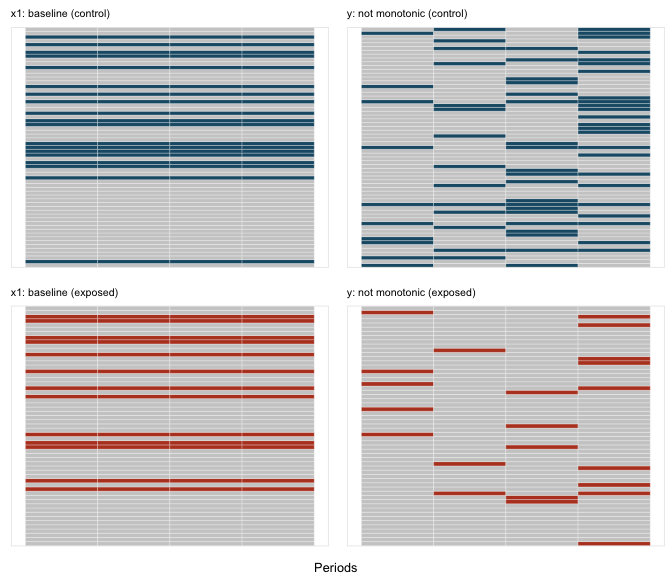In the second case, missingness is monotonic; once a subject misses a measurement for y, there are no subsequent measurements: # missingness for y is not monotonic defMlong <- defMiss(varname = "x1", formula = 0.2, baseline = TRUE) defMlong <- defMiss(defMlong, varname = "y", formula = "-1.8 - 1.5 * rx + .25*period", logit.link = TRUE, baseline = FALSE, monotonic = TRUE) missMatLong <- genMiss(dtName = dtTime, missDefs = defMlong, idvars = c("id", "rx"), repeated = TRUE, periodvar = "period")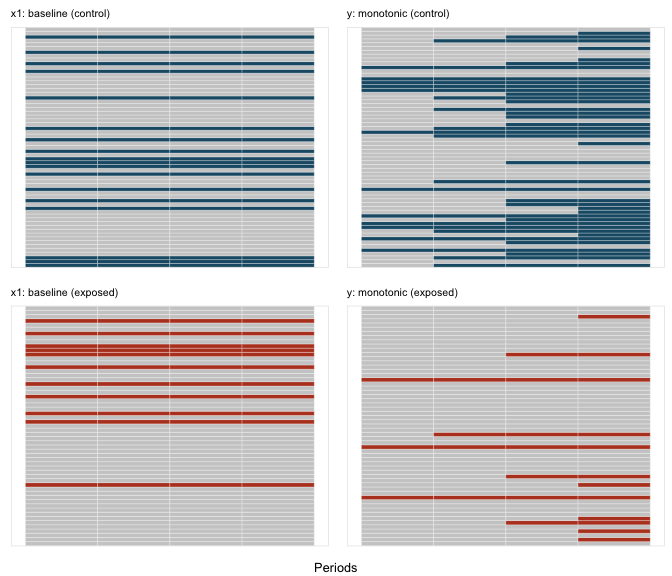## Spline data Sometimes (usually?) relationships between variables are non-linear. simstudy can already accommodate that. But, if we want to explicitly generate data from a piece-wise polynomial function to explore spline methods in particular, or non-linear relationships more generally. There are three functions that facilitate this: viewBasis, viewSplines, and genSpline. The first two functions are more exploratory in nature, and just provide plots of the B-spline basis functions and the splines, respectively. The third function actually generates data and adds to an existing data.table. The shape of a spline is determined by three factors: (1) the cut-points or knots that define the piecewise structure of the function, (2) the polynomial degree, such as linear, quadratic, cubic, etc., and (3) the linear coefficients that combine the basis functions, which is contained in a vector or matrix theta. First, we can look at the basis functions, we depend only the knots and degree. The knots are specified as quantiles, between 0 and 1: knots <- c(0.25, 0.5, 0.75) viewBasis(knots, degree = 2)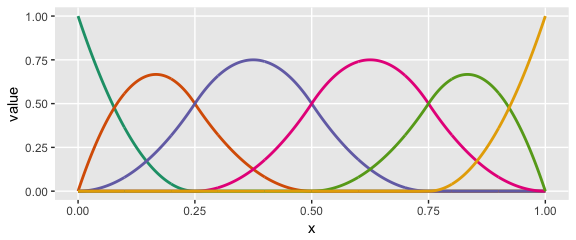knots <- c(0.2, 0.4, 0.6, 0.8) viewBasis(knots, degree = 3)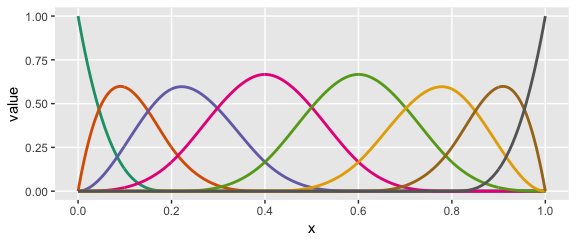The splines themselves are specified as linear combinations of each of the basis functions. The coefficients of those combinations are specified in theta. Each individual spline curve represents a specific linear combination of a particular set of basis functions. In exploring, we can look at a single curve or multiple curves, depending on whether or not we specify theta as a vector (single) or matrix (multiple). knots <- c(0.25, 0.5, 0.75) # number of elements in theta: length(knots) + degree + 1 theta1 = c(0.1, 0.8, 0.4, 0.9, 0.2, 1) viewSplines(knots, degree = 2, theta1)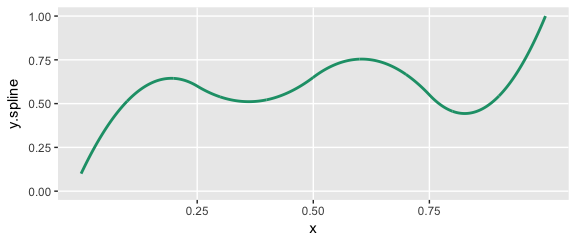theta2 = matrix(c(0.1, 0.2, 0.4, 0.9, 0.2, 0.3, 0.6, 0.1, 0.3, 0.3, 0.8, 1, 0.9, 0.4, 0.1, 0.9, 0.8, 0.2, 0.1, 0.6, 0.1), ncol = 3) theta2 ## [,1] [,2] [,3] ## [1,] 0.1 0.1 0.1 ## [2,] 0.2 0.3 0.9 ## [3,] 0.4 0.3 0.8 ## [4,] 0.9 0.8 0.2 ## [5,] 0.2 1.0 0.1 ## [6,] 0.3 0.9 0.6 ## [7,] 0.6 0.4 0.1 viewSplines(knots, degree = 3, theta2)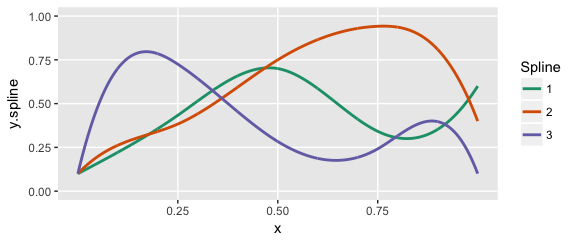We can generate data using a predictor in an existing data set by specifying the knots (in terms of quantiles), a vector of coefficients in theta, the degree of polynomial, as well as a range ddef <- defData(varname = "age", formula = "20;60", dist = "uniform") theta1 = c(0.1, 0.8, 0.6, 0.4, 0.6, 0.9, 0.9) knots <- c(0.25, 0.5, 0.75) Here is the shape of the curve that we want to generate data from: viewSplines(knots = knots, theta = theta1, degree = 3)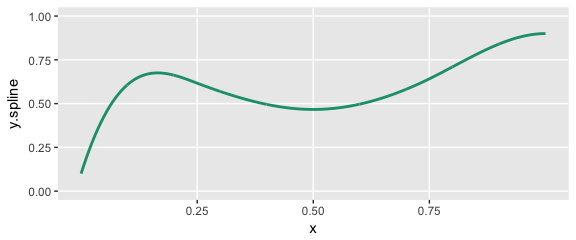Now we specify the variables in the data set and generate the data: set.seed(234) dt <- genData(1000, ddef) dt <- genSpline(dt = dt, newvar = "weight", predictor = "age", theta = theta1, knots = knots, degree = 3, newrange = "90;160", noise.var = 64) Here’s a plot of the data with a smoothed line fit to the data: ggplot(data = dt, aes(x = age, y = weight)) + geom_point(color = "grey65", size = 0.75) + geom_smooth(se = FALSE, color = "red", size = 1, method = "auto") + geom_vline(xintercept = quantile(dt$age,
knots)) + theme(panel.grid.minor = element_blank())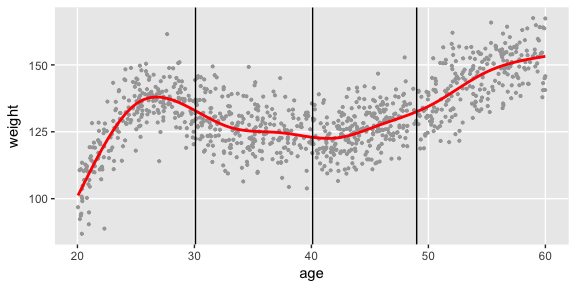Finally, we will fit three different spline models to the data - a linear, a quadratic, and a cubic - and plot the predicted values:

# normalize age for best basis functions
dt[, :=(nage, (age - min(age))/(max(age) - min(age)))]

# fit a cubic spline
lmfit3 <- lm(weight ~ bs(x = nage, knots = knots, degree = 3, intercept = TRUE) -
1, data = dt)

lmfit2 <- lm(weight ~ bs(x = nage, knots = knots, degree = 2), data = dt)

# fit a linear spline
lmfit1 <- lm(weight ~ bs(x = nage, knots = knots, degree = 1), data = dt)

# add predicted values for plotting
dt[, :=(pred.3deg, predict(lmfit3))]
dt[, :=(pred.2deg, predict(lmfit2))]
dt[, :=(pred.1deg, predict(lmfit1))]

ggplot(data = dt, aes(x = age, y = weight)) + geom_point(color = "grey65", size = 0.75) +
geom_line(aes(x = age, y = pred.3deg), color = "#1B9E77", size = 1) + geom_line(aes(x = age,
y = pred.2deg), color = "#D95F02", size = 1) + geom_line(aes(x = age, y = pred.1deg),
color = "#7570B3", size = 1) + geom_vline(xintercept = quantile(dt\$age,
knots)) + theme(panel.grid.minor = element_blank())